【一猫汽车网常州站】众所周知，排气分为头段、中段和尾段，往往中尾段是广大车友升级爱车的必备硬件，殊不知头段的升级改装反而对性能和操控的提升更加的明显。我们所说的头段指的是三元催化这一段，三元催化主要就是通过自身的载体起到净化尾气的作用，三元催化过滤了尾气的同时也限制了排气的速率和流量，从而限制了发动机的输出功率往往表现为轮上的功率和发动机功率落差巨大。为了给广大性能爱好者解决这一问题，K2 MOTOR工程师历经数年辛苦钻研和不断的尝试，终于用一根钢管解决了所有的问题。于是 K2 MOTOR 直通型头段横空而出，赋予一根钢管新的生命和价值定义。发布会合影

K2 MOTOR直通型头段于2015年初正式从检测室走出，经过严苛的质量检测、路试检测、客户试用反馈调查及性能提升检测后，于2015年下半年正式量产，先期产品仅限美国本土销售，以便于更好的获取产品反馈信息。产品在美国本土上市后一鸣惊人、销量节节攀升。直通头段粗管径、大功率、原装位设计与美国人的粗犷、豪迈完美融合，让广大美国消费者爱不释手。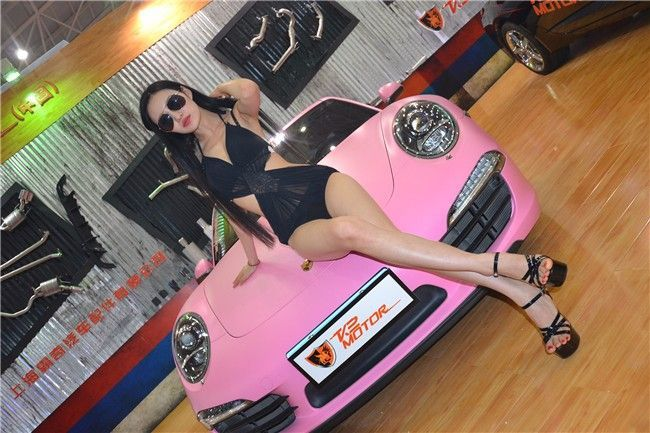K2排气新品发布

K2 MOTOR(中国)看到了该产品在国内市场的销售前景，于2015年年底开始小批量引进，主要做一些测试、试装、试用的工作，为后来批量引进打下牢固的基础。K2 MOTOR直通型头段采用优质304不锈钢管作为原料，采用粗管径、大功率、原装位设计，法兰采用大型CNC数控机床加工而成，采用加厚加固原装位设计确保牢固可靠，产品表面采用钛合金工艺处理，让产品美观时尚质感诱人。头段内加装了独一无二的读取尾气信号装置，能准确的让车辆电脑读取空油比数据，让发动机喷油、点火、电控装置正常无误的工作，并确保不亮故障灯。产品安装后能有效提升排气的速率，加大排气流量，从而进一步提升车辆动力同时还可以让排气声浪更加低沉、浑厚;更能有效提升涡轮车的散热速度，提高涡轮使用寿命;搭配进气设备、电脑外挂或程序同时使用其效果更加。经过长期持续的检测和测试后K2 MOTOR于2016年初开始批量引进，并在2016年6月份的武汉UTS改装车展上发布该款产品，于是这根钢管就漂洋过海从洛杉矶来到了武汉。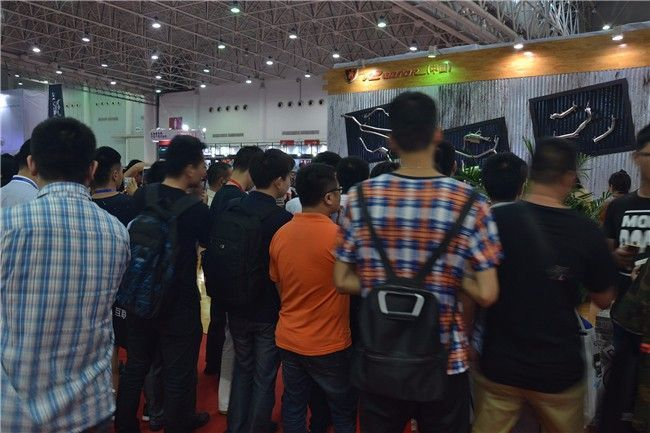2016武汉UTS K2 MOTOR新品发布现场人气爆棚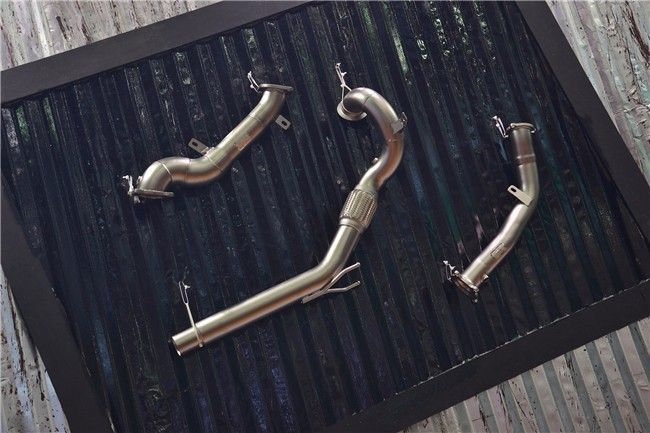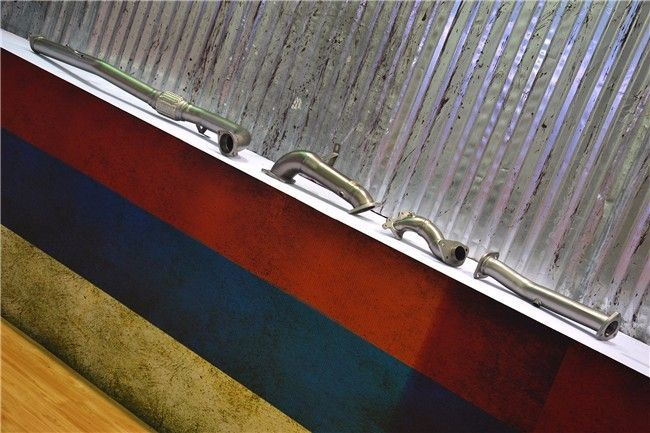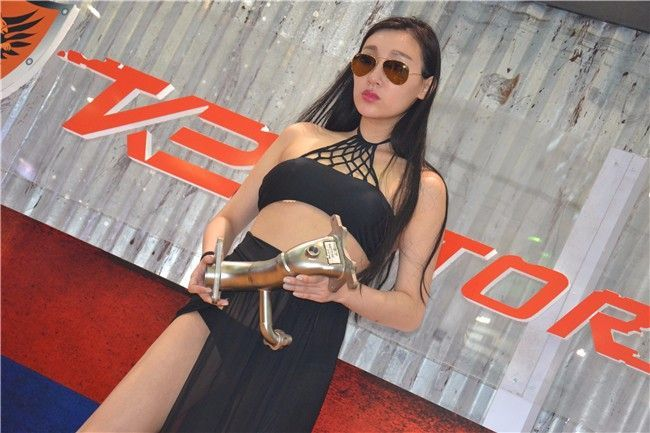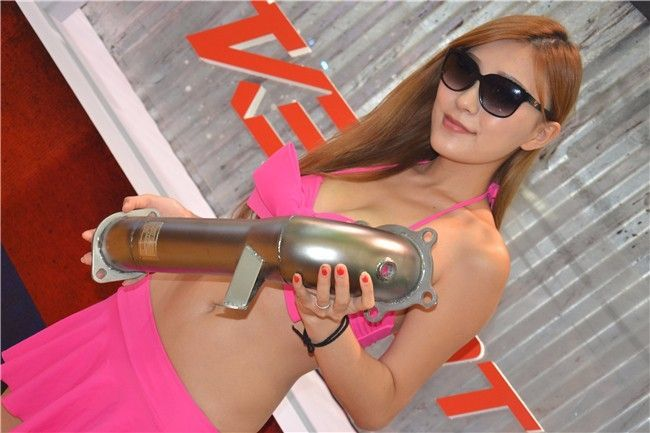模特手持头段新品走秀-发布现场在这样的氛围下这根钢管开始了它在武汉的梦幻旅行，和我们一起参观的武汉的大江、大湖和“大海”，与美丽大方的模特们一起亲密接触，与现场的观众互动游戏，分享精美的礼品，乐不思蜀。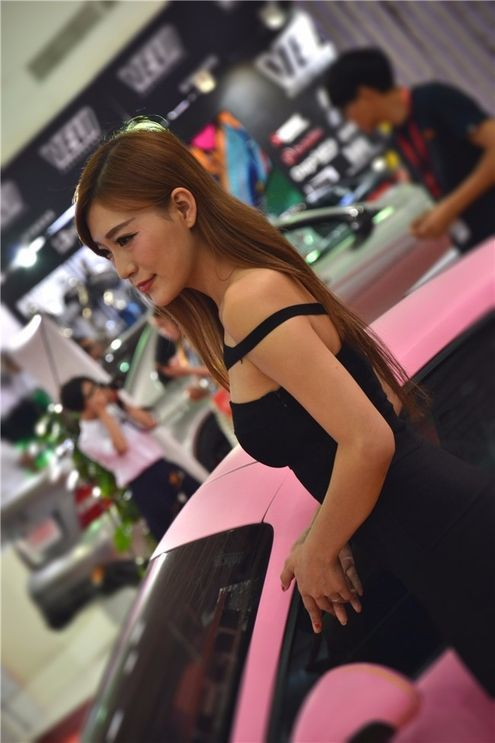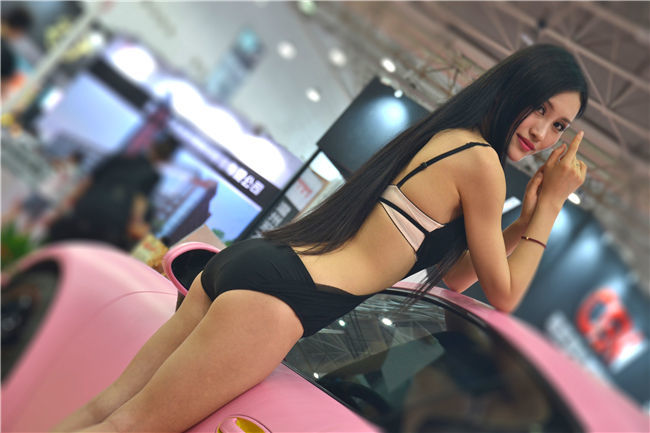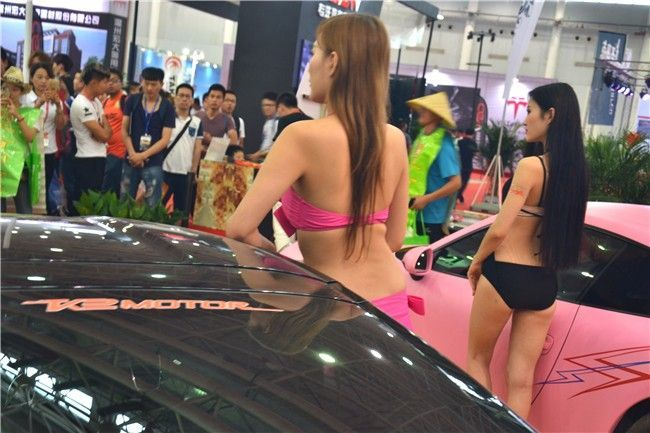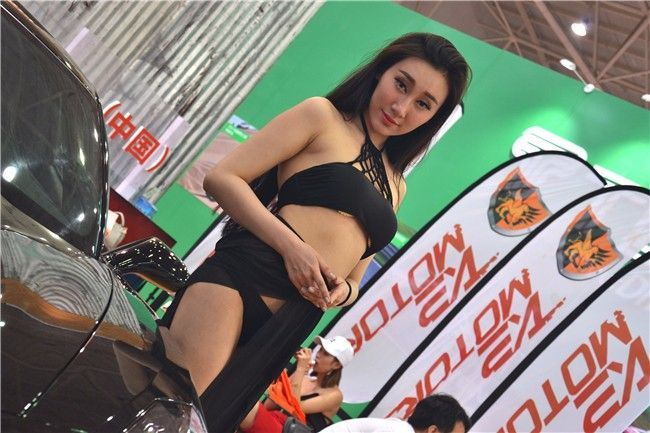随着武汉UTS改装车展的落幕，这根钢管的旅行似乎就此结束了，事实上它的旅途仅仅是一个开始而已。自武汉亮相以来它的足迹遍及中国各地，它以优良的品质、稳定的性能、独有的价值功能备受各地消费者的青睐，各地经销商签约不断。

奔驰A200直通头段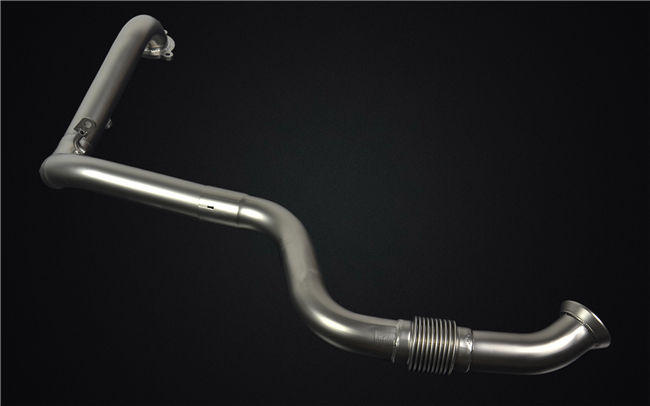凯迪拉克ATS直通头段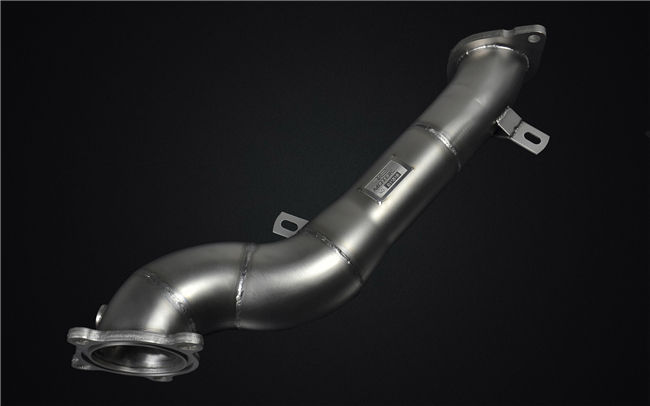飞度GK5直通头段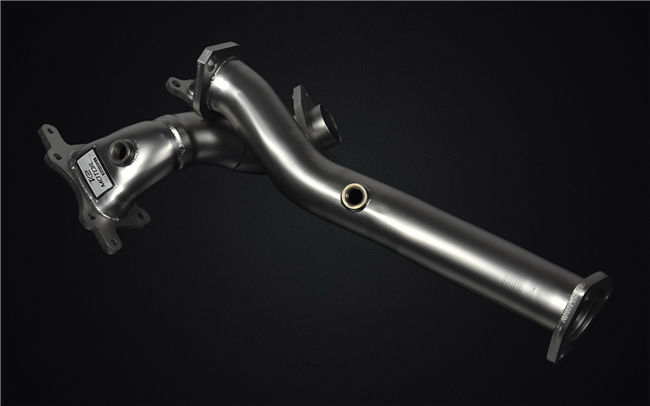高尔夫GTI直通头段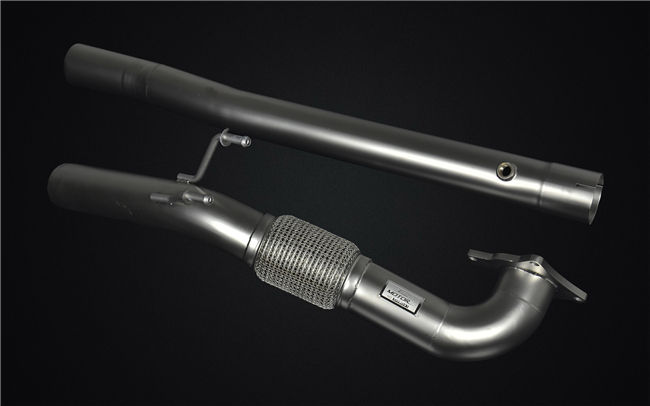宝马M3直通头段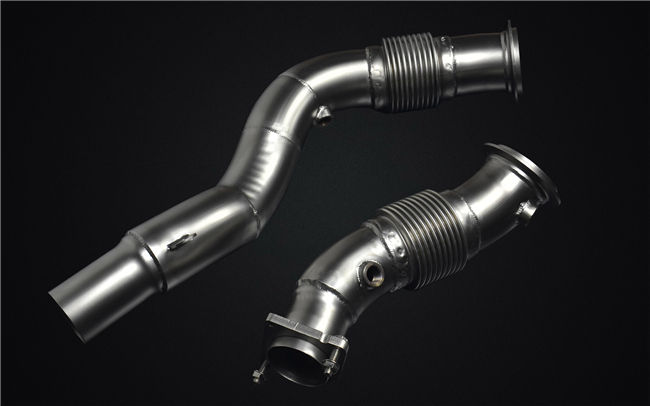英菲尼迪Q50直通头段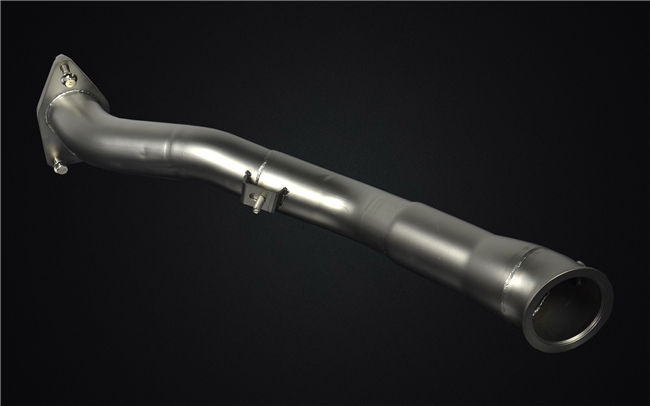凯迪拉克XTS直通头段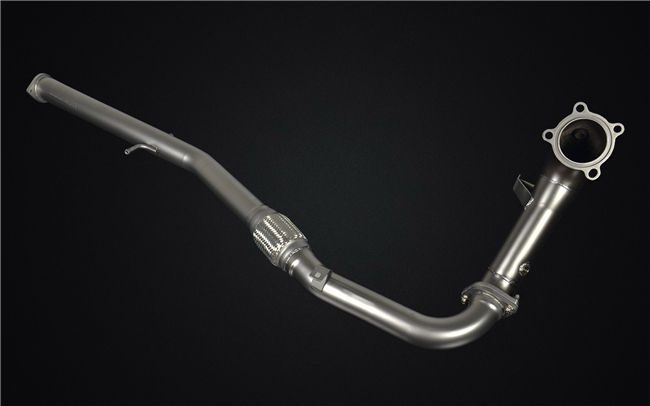随着国内汽车改装文化的不断发展，越来越多的改装爱好者开始正确、理性的选择改装产品。排气升级也从开始的中尾段发展为全段排气，大家关注的不仅仅是排气的外观、排气的声浪，而且对排气的性能指标参数也有新的要求。其实对于排气改装来说，头段改装带来的性能提升、动力提升、声浪提升都是大于只改中尾段的，我们一直看好该产品在中国市场的销售前景。K2 MOTOR工程师将一如既往的推陈出新，努力推出更多性能卓越的产品以满足广大消费者的不同需求。K2 MOTOR直通头段现已大量大货，产品覆盖多个车型、库存充足发货及时，需要了解的可以通过以下方式咨询。

|分享

•已阅！握爪
•我手滑为你点赞
•128个赞！
•不明觉厉
•阅后既醉
•有钱！任性
•照片太美，我不敢看
•干货！杠杠的
•高大上
•膜拜中！
•请接受我的膝盖
•猫哥/猫妹么么哒
•神吐槽
•我只笑笑不说话
•我想静静
•窒息
##### 热度排行• 阿斯顿·马丁
• 奥迪
• 阿尔法罗密欧
• ALPINA
• 爱驰
• AC Schnitzer
• AITO
• ARCFOX极狐
• AUXUN傲旋

• 巴博斯
• 宝骏
• 宝马
• 保时捷
• 北汽制造
• 奔驰
• 奔腾
• 本田
• 比亚迪
• 标致
• 别克
• 宾利
• 布加迪
• 北汽威旺
• 北京
• 北汽绅宝
• 北汽幻速
• 北汽新能源
• 宝沃
• 比速汽车
• 北汽道达
• 比德文汽车
• 铂驰
• 北汽昌河
• 百智新能源
• 宝骐汽车
• 比克汽车
• 博速

• 昌河
• 长安
• 长城
• 长安商用
• 成功汽车
• 长江EV
• 长安轻车型
• 长安欧尚
• 长安凯程
• 车驰汽车
• 橙仕
• 创维汽车

• 大众
• 道奇
• 东风
• 东风风神
• 东风小康
• 东南
• DS
• 东风风行
• 东风风度
• 东风风光
• 电咖
• 大乘汽车
• 大运
• 东风富康
• 东风·瑞泰特
• 东风新能源
• 东风御风

• 法拉利
• 菲亚特
• 丰田
• 福迪
• 福特
• 福田汽车
• 福汽启腾
• 风诺
• 飞碟汽车
• 飞凡汽车

• GMC
• 广汽传祺
• 广汽吉奥
• 观致
• 国金汽车
• 国机智骏
• 高合汽车
• 广汽埃安

• 哈飞
• 海格
• 海马
• 华泰
• 黄海
• 恒天
• 红旗
• 哈弗
• 华颂
• 华凯
• 华泰新能源
• 汉腾汽车
• 华利
• 红星汽车
• HYCAN合创
• 汉龙汽车
• 恒润汽车
• 宏远汽车
• 华晨新日
• 华骐
• 华梓汽车

• Icona
• IMSA英飒

• Jeep
• 江淮
• 江铃
• 捷豹
• 金杯
• 九龙
• 吉利汽车
• 金旅
• 金龙
• 江铃集团轻汽
• 江铃集团新能源
• 君马汽车
• 捷途
• 钧天
• 捷达
• 几何汽车
• 极氪
• 捷尼赛思
• 金冠汽车

• 开瑞
• 凯迪拉克
• 科尼赛克
• 克莱斯勒
• KTM
• 卡威
• 凯翼
• 康迪
• 康迪电动汽车集团
• 开沃汽车
• 卡升
• 克蒂汽车
• 克慕勒

• 猎豹汽车
• 兰博基尼
• 劳斯莱斯
• 雷克萨斯
• 雷诺
• 理念
• 力帆
• 莲花汽车
• 林肯
• 铃木
• 陆风
• 路虎
• 路特斯
• 领志
• 领克
• 零跑汽车
• Lorinser
• LOCAL MOTORS
• 领途汽车
• 理想汽车
• 罗夫哈特
• 力帆汽车
• LEVC
• LIMGENE凌际
• LUMMA
• 莱茵汽车
• 岚图汽车
• 凌宝汽车
• 龙程汽车

• MG
• MINI
• 马自达
• 玛莎拉蒂
• 迈凯伦
• 摩根
• 迈莎锐
• 迈迈
• 摩登汽车

• 纳智捷
• 哪吒汽车

• 讴歌
• 欧宝
• 欧朗
• 欧拉
• 欧尚汽车

• 帕加尼
• Polestar极星
• 朋克汽车

• 奇瑞
• 启辰
• 起亚
• 前途
• 庆铃汽车
• 骐铃汽车
• 乔治·巴顿

• 日产
• 荣威
• 瑞麒汽车
• 如虎
• 瑞驰
• 瑞驰新能源
• 容大智造
• RAM
• 睿蓝汽车

### S

• 上汽大通
• smart
• 三菱
• 双环
• 双龙
• 斯巴鲁
• 斯柯达
• 萨博
• 思铭
• 赛麟
• SWM斯威汽车
• SRM鑫源
• 思皓
• SHELBY
• 上喆
• SERES赛力斯
• SONGSAN MOTORS
• 速达
• 申龙客车
• 神州

• TESLA
• 泰卡特
• 腾势
• 特斯拉
• 天际汽车
• TECHART
• 坦克

• 威麟
• 威兹曼
• 沃尔沃
• 五菱汽车
• 五十铃
• 潍柴英致
• WEY
• 蔚来
• 威马汽车
• WALD
• 瓦滋
• 伟昊汽车
• 魏牌

• 现代
• 雪佛兰
• 雪铁龙
• 西雅特
• 新特汽车
• 小鹏汽车
• 新宝骏
• 小虎
• 小猬汽车
• 晓奥汽车

• 野马汽车
• 一汽
• 依维柯
• 英菲尼迪
• 永源
• 驭胜
• 云度
• 宇通客车
• 云雀汽车
• 远程汽车
• 一汽凌河
• 雅升汽车
• 野马新能源
• 银隆新能源
• 御捷
• 裕路

• 中华
• 中兴
• 众泰
• 知豆
• 之诺
• 正道汽车
• 智点汽车
• 智己汽车
• 中国重汽VGV
• A
• B
• C
• D
• E
• F
• G
• H
• I
• J
• K
• L
• M
• N
• O
• P
• Q
• R
• S
• T
• U
• V
• W
• X
• Y
• Z

• 阿斯顿·马丁
• 奥迪
• 阿尔法罗密欧
• ALPINA
• 爱驰
• AC Schnitzer
• AITO
• ARCFOX极狐
• AUXUN傲旋

• 巴博斯
• 宝骏
• 宝马
• 保时捷
• 北汽制造
• 奔驰
• 奔腾
• 本田
• 比亚迪
• 标致
• 别克
• 宾利
• 布加迪
• 北汽威旺
• 北京
• 北汽绅宝
• 北汽幻速
• 北汽新能源
• 宝沃
• 比速汽车
• 北汽道达
• 比德文汽车
• 铂驰
• 北汽昌河
• 百智新能源
• 宝骐汽车
• 比克汽车
• 博速

• 昌河
• 长安
• 长城
• 长安商用
• 成功汽车
• 长江EV
• 长安轻车型
• 长安欧尚
• 长安凯程
• 车驰汽车
• 橙仕
• 创维汽车

• 大众
• 道奇
• 东风
• 东风风神
• 东风小康
• 东南
• DS
• 东风风行
• 东风风度
• 东风风光
• 电咖
• 大乘汽车
• 大运
• 东风富康
• 东风·瑞泰特
• 东风新能源
• 东风御风

• 法拉利
• 菲亚特
• 丰田
• 福迪
• 福特
• 福田汽车
• 福汽启腾
• 风诺
• 飞碟汽车
• 飞凡汽车

• GMC
• 广汽传祺
• 广汽吉奥
• 观致
• 国金汽车
• 国机智骏
• 高合汽车
• 广汽埃安

• 哈飞
• 海格
• 海马
• 华泰
• 黄海
• 恒天
• 红旗
• 哈弗
• 华颂
• 华凯
• 华泰新能源
• 汉腾汽车
• 华利
• 红星汽车
• HYCAN合创
• 汉龙汽车
• 恒润汽车
• 宏远汽车
• 华晨新日
• 华骐
• 华梓汽车

• Icona
• IMSA英飒

• Jeep
• 江淮
• 江铃
• 捷豹
• 金杯
• 九龙
• 吉利汽车
• 金旅
• 金龙
• 江铃集团轻汽
• 江铃集团新能源
• 君马汽车
• 捷途
• 钧天
• 捷达
• 几何汽车
• 极氪
• 捷尼赛思
• 金冠汽车

• 开瑞
• 凯迪拉克
• 科尼赛克
• 克莱斯勒
• KTM
• 卡威
• 凯翼
• 康迪
• 康迪电动汽车集团
• 开沃汽车
• 卡升
• 克蒂汽车
• 克慕勒

• 猎豹汽车
• 兰博基尼
• 劳斯莱斯
• 雷克萨斯
• 雷诺
• 理念
• 力帆
• 莲花汽车
• 林肯
• 铃木
• 陆风
• 路虎
• 路特斯
• 领志
• 领克
• 零跑汽车
• Lorinser
• LOCAL MOTORS
• 领途汽车
• 理想汽车
• 罗夫哈特
• 力帆汽车
• LEVC
• LIMGENE凌际
• LUMMA
• 莱茵汽车
• 岚图汽车
• 凌宝汽车
• 龙程汽车

• MG
• MINI
• 马自达
• 玛莎拉蒂
• 迈凯伦
• 摩根
• 迈莎锐
• 迈迈
• 摩登汽车

• 纳智捷
• 哪吒汽车

• 讴歌
• 欧宝
• 欧朗
• 欧拉
• 欧尚汽车

• 帕加尼
• Polestar极星
• 朋克汽车

• 奇瑞
• 启辰
• 起亚
• 前途
• 庆铃汽车
• 骐铃汽车
• 乔治·巴顿

• 日产
• 荣威
• 瑞麒汽车
• 如虎
• 瑞驰
• 瑞驰新能源
• 容大智造
• RAM
• 睿蓝汽车

### S

• 上汽大通
• smart
• 三菱
• 双环
• 双龙
• 斯巴鲁
• 斯柯达
• 萨博
• 思铭
• 赛麟
• SWM斯威汽车
• SRM鑫源
• 思皓
• SHELBY
• 上喆
• SERES赛力斯
• SONGSAN MOTORS
• 速达
• 申龙客车
• 神州

• TESLA
• 泰卡特
• 腾势
• 特斯拉
• 天际汽车
• TECHART
• 坦克

• 威麟
• 威兹曼
• 沃尔沃
• 五菱汽车
• 五十铃
• 潍柴英致
• WEY
• 蔚来
• 威马汽车
• WALD
• 瓦滋
• 伟昊汽车
• 魏牌

• 现代
• 雪佛兰
• 雪铁龙
• 西雅特
• 新特汽车
• 小鹏汽车
• 新宝骏
• 小虎
• 小猬汽车
• 晓奥汽车

• 野马汽车
• 一汽
• 依维柯
• 英菲尼迪
• 永源
• 驭胜
• 云度
• 宇通客车
• 云雀汽车
• 远程汽车
• 一汽凌河
• 雅升汽车
• 野马新能源
• 银隆新能源
• 御捷
• 裕路

• 中华
• 中兴
• 众泰
• 知豆
• 之诺
• 正道汽车
• 智点汽车
• 智己汽车
• 中国重汽VGV
• A
• B
• C
• D
• E
• F
• G
• H
• I
• J
• K
• L
• M
• N
• O
• P
• Q
• R
• S
• T
• U
• V
• W
• X
• Y
• Z

• 阿斯顿·马丁
• 奥迪
• 阿尔法罗密欧
• ALPINA
• 爱驰
• AC Schnitzer
• AITO
• ARCFOX极狐
• AUXUN傲旋

• 巴博斯
• 宝骏
• 宝马
• 保时捷
• 北汽制造
• 奔驰
• 奔腾
• 本田
• 比亚迪
• 标致
• 别克
• 宾利
• 布加迪
• 北汽威旺
• 北京
• 北汽绅宝
• 北汽幻速
• 北汽新能源
• 宝沃
• 比速汽车
• 北汽道达
• 比德文汽车
• 铂驰
• 北汽昌河
• 百智新能源
• 宝骐汽车
• 比克汽车
• 博速

• 昌河
• 长安
• 长城
• 长安商用
• 成功汽车
• 长江EV
• 长安轻车型
• 长安欧尚
• 长安凯程
• 车驰汽车
• 橙仕
• 创维汽车

• 大众
• 道奇
• 东风
• 东风风神
• 东风小康
• 东南
• DS
• 东风风行
• 东风风度
• 东风风光
• 电咖
• 大乘汽车
• 大运
• 东风富康
• 东风·瑞泰特
• 东风新能源
• 东风御风

• 法拉利
• 菲亚特
• 丰田
• 福迪
• 福特
• 福田汽车
• 福汽启腾
• 风诺
• 飞碟汽车
• 飞凡汽车

• GMC
• 广汽传祺
• 广汽吉奥
• 观致
• 国金汽车
• 国机智骏
• 高合汽车
• 广汽埃安

• 哈飞
• 海格
• 海马
• 华泰
• 黄海
• 恒天
• 红旗
• 哈弗
• 华颂
• 华凯
• 华泰新能源
• 汉腾汽车
• 华利
• 红星汽车
• HYCAN合创
• 汉龙汽车
• 恒润汽车
• 宏远汽车
• 华晨新日
• 华骐
• 华梓汽车

• Icona
• IMSA英飒

• Jeep
• 江淮
• 江铃
• 捷豹
• 金杯
• 九龙
• 吉利汽车
• 金旅
• 金龙
• 江铃集团轻汽
• 江铃集团新能源
• 君马汽车
• 捷途
• 钧天
• 捷达
• 几何汽车
• 极氪
• 捷尼赛思
• 金冠汽车

• 开瑞
• 凯迪拉克
• 科尼赛克
• 克莱斯勒
• KTM
• 卡威
• 凯翼
• 康迪
• 康迪电动汽车集团
• 开沃汽车
• 卡升
• 克蒂汽车
• 克慕勒

• 猎豹汽车
• 兰博基尼
• 劳斯莱斯
• 雷克萨斯
• 雷诺
• 理念
• 力帆
• 莲花汽车
• 林肯
• 铃木
• 陆风
• 路虎
• 路特斯
• 领志
• 领克
• 零跑汽车
• Lorinser
• LOCAL MOTORS
• 领途汽车
• 理想汽车
• 罗夫哈特
• 力帆汽车
• LEVC
• LIMGENE凌际
• LUMMA
• 莱茵汽车
• 岚图汽车
• 凌宝汽车
• 龙程汽车

• MG
• MINI
• 马自达
• 玛莎拉蒂
• 迈凯伦
• 摩根
• 迈莎锐
• 迈迈
• 摩登汽车

• 纳智捷
• 哪吒汽车

• 讴歌
• 欧宝
• 欧朗
• 欧拉
• 欧尚汽车

• 帕加尼
• Polestar极星
• 朋克汽车

• 奇瑞
• 启辰
• 起亚
• 前途
• 庆铃汽车
• 骐铃汽车
• 乔治·巴顿

• 日产
• 荣威
• 瑞麒汽车
• 如虎
• 瑞驰
• 瑞驰新能源
• 容大智造
• RAM
• 睿蓝汽车

### S

• 上汽大通
• smart
• 三菱
• 双环
• 双龙
• 斯巴鲁
• 斯柯达
• 萨博
• 思铭
• 赛麟
• SWM斯威汽车
• SRM鑫源
• 思皓
• SHELBY
• 上喆
• SERES赛力斯
• SONGSAN MOTORS
• 速达
• 申龙客车
• 神州

• TESLA
• 泰卡特
• 腾势
• 特斯拉
• 天际汽车
• TECHART
• 坦克

• 威麟
• 威兹曼
• 沃尔沃
• 五菱汽车
• 五十铃
• 潍柴英致
• WEY
• 蔚来
• 威马汽车
• WALD
• 瓦滋
• 伟昊汽车
• 魏牌

• 现代
• 雪佛兰
• 雪铁龙
• 西雅特
• 新特汽车
• 小鹏汽车
• 新宝骏
• 小虎
• 小猬汽车
• 晓奥汽车

• 野马汽车
• 一汽
• 依维柯
• 英菲尼迪
• 永源
• 驭胜
• 云度
• 宇通客车
• 云雀汽车
• 远程汽车
• 一汽凌河
• 雅升汽车
• 野马新能源
• 银隆新能源
• 御捷
• 裕路

• 中华
• 中兴
• 众泰
• 知豆
• 之诺
• 正道汽车
• 智点汽车
• 智己汽车
• 中国重汽VGV
• A
• B
• C
• D
• E
• F
• G
• H
• I
• J
• K
• L
• M
• N
• O
• P
• Q
• R
• S
• T
• U
• V
• W
• X
• Y
• Z